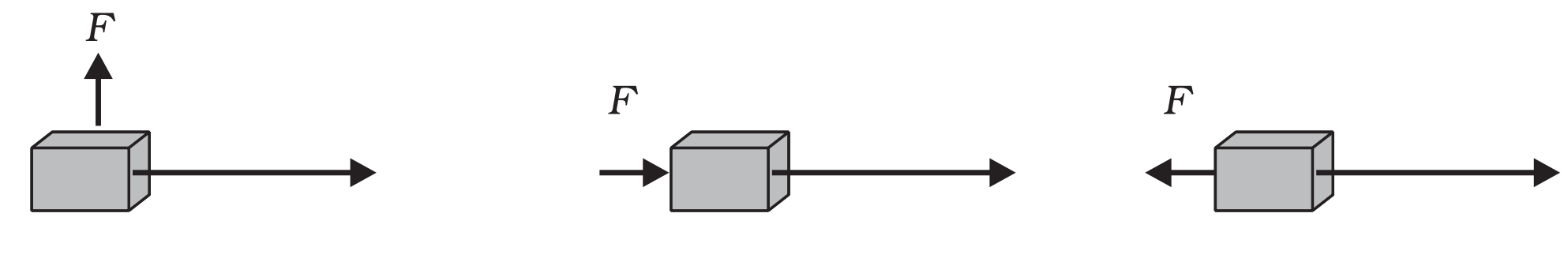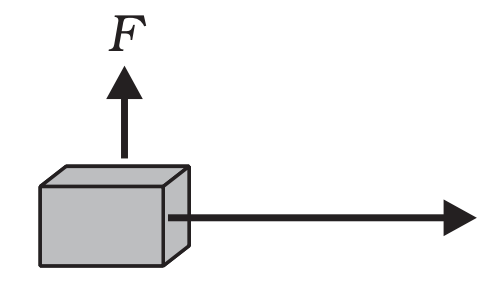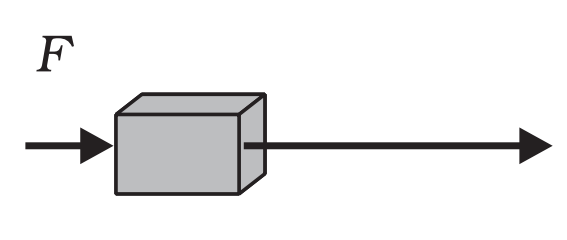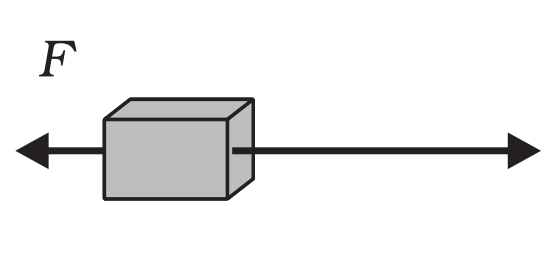NCERT Questions

Class 9
Chapter 11 Class 9 - Work and Energy

## In each of the following a force, F is acting on an object of mass, m. The direction of displacement is from west to east shown by the longer arrow. Observe the diagrams carefully and state whether the work done by the force is negative, positive or zeroWe know that

Work done = Force × Displacement × cos θ

Where, theta is the angle between the force and the displacement

Case1

###(adsbygoogle = window.adsbygoogle || []).push({});

The angle between the force and displacement is 90 degrees.

Work done = Force × Displacement × cos θ

= Force × Displacement × cos 90

= Force × Displacement × 0

= 0

Hence, work done is 0 .

Case 2

###The angle between the force and displacement is 0 degrees.

Work done = Force × Displacement × cos θ

= Force × Displacement × cos 0

= Force × Displacement × 1

= Force × Displacement

Hence, work done is positive .

Case 3

###The angle between the force and displacement is 180 degrees.

Work done = Force × Displacement × cos θ

= Force × Displacement × cos 18 0

= Force × Displacement × (-1)

= - Force × Displacement

Hence, the work done is negative.

Learn in your speed, with individual attention - Teachoo Maths 1-on-1 Class